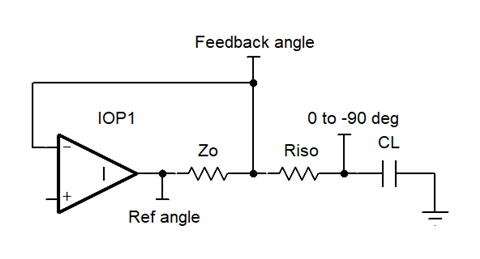If you have a related question, please click the "Ask a related question" button in the top right corner. The newly created question will be automatically linked to this question.

• TI Thinks Resolved

# LMV881: R_ISO

Part Number: LMV881

Hi,

Page 14 of the datasheet (see table under Figure 29) shows that as the capacitive load increases, the isolation resistor is decreasing - is this correct and how is this calculated?

Thanks,

Chuchen

• In reply to kai klaas69:

Hello Chuchen,

We have several resources on this topic. The first is a TI Design (TIPD) that discusses the issue in depth. The second resource is a TI Precision Labs Op Amp Video/Slides set explaining the Riso method for maintaining stability with larger capacitive loads. Both of these describe the theory behind the Riso method and the methodology for selecting the isolation resistor value. This should answer the second part of your question.

TIPD 128: http://www.ti.com/tool/TIPD128
TI Precision Labs on Riso: training.ti.com/ti-precision-labs-op-amps-stability-5

To summarize these resources, the issue with a large capacitive load is instability caused by the low frequency output pole from the large capacitance load. The purpose of adding an isolation resistor is to effectively cancel out the output pole by adding a zero at a nearby frequency, giving us an effective pole-zero pair. This is the basis for the Riso calculation.

To answer the first part of your question: yes. It is normal for the minimum isolation resistor to decrease as the load impedance increases, though this might seem counterintuitive (see pages 21 - 25 of TIPD 128 User Guide). You can still use a larger resistor if you'd like, but it is not necessary and will lead to a larger output voltage offset. We pick the isolation resistor to be a small as possible to minimize this offset while maintaining a sufficient amount of stability.

The minimum isolation resistance decreases as the load capacitance increases because, as you increase the load capacitance but maintain the isolation resistor, the pole-zero pair occurs at a lower frequency range. Consequently, there is more room for the phase to correct itself and reach an acceptable phase margin before crossing 1/Beta (0 dB for unity buffer configuration) as the zero frequency comes in earlier. Thus, a smaller value isolation resistor can be allowed to sacrifice a bit of phase margin for output offset voltage.

This might be a bit convoluted, so let me know if anything is unclear.

Regards,
Daniel
• In reply to Daniel Miller56:

Chuchen,

Here is a simple way to look at it. I'll make up some numbers in the text and assume Zo is resistive.The LMV881 is unity gain stable by itself. CL=0pF. No load means feedback angle (phase lag) is same as the reference angle. At lower (not lowest) frequencies the Ref angle is -90 degrees as op amp gain is dropping with frequency (-20db/decade). By the bandwidth frequency for this device where gain drops to is 0dB, Ref angle could be down to -130 degrees relative to input. (Phase Margin is 50 degrees, good stability)

Now we add Riso and CL. Notice Zo (op amp output impedance) and Riso makes a voltage divider (and phase lag divider) therefore the lag at the feedback is less than the lag at the capacitor (up to - 90 degrees relative to the ref angle). The feedback angle varies between ref angle and cap angle based on the Zo , Riso ratio whether a zero or pole occurred or not.

The the higher the capacitance the lower the bandwidth of the op amp loop will be. Any cap load that reduces bandwidth down to frequencies where the op amp lag (ref angle) is -90 degrees will be easy to stabilize with Riso because we get (-90 - -130) 40 degrees more margin to use. With op amp at -90 degrees and cap at -90 degrees any phase relief at feedback node from Riso becomes phase margin.

Oddly enough this is a simple explanation. If Zo is not resistive then things get much more complicated.

Regards,
Ronald Michallick
Linear Applications

TI assumes no liability for applications assistance or customer product design. Customer is fully responsible for all design decisions and engineering with regard to its products, including decisions relating to application of TI products. By providing technical information, TI does not intend to offer or provide engineering services or advice concerning Customer's design. If Customer desires engineering services, the Customer should rely on its retained employees and consultants and/or procure engineering services from a licensed professional engineer (LPE).